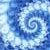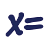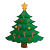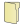Games
Problems
Go Pro!

# Number and Quantity

Pro Problems > Math > Number and Quantity

# Featured Pro ProblemsWithout a calculator find a nine digit number that meets the criteria of the problemA math problem involving Shrek wanting Fiona's telephone numberMultiply a three by one matrix and a one by three matrixStrings of red lights and green lights on my Christmas tree - a Christmas word problemSolve for x in this matrix multiplication equationFind the value of w in the matrix multiplication problemA diophantine equation - quadratic in two variablesUse divisibility rules to determine the number with missing digits# Full Directory Listing

The Value of a Word, Trick-or-Treat Math, Two NumbersLike us on Facebook to get updates about new resources